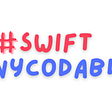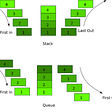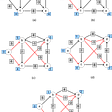# Question Overview

You are given an integer array `nums`, find the contiguous subarray (containing at least one number) which has the largest sum and return its sum.

# Example

Input: [-2,1,-3,4,-1,2,1,-5,4],
Output: 6
Explanation: [4,-1,2,1] has the largest sum = 6.

Assume nums.length=1, the largest sum will be nums.

Assume num.length=2, the largest num will be either num or num+num, we can use max method to determine which one is bigger. If the nums < nums + nums, replace the largest sum with the sum of the first two elements. store the value of the largest sum to compare with the sum of the first three element, and so on and so forth.

I started by defining two variables, largest_sum and current.

`largest_sum = numscurrent = 0`

Next, loop through the array with each enumerable.

`nums.each {|n| largest_sum = [largest_sum, current = [n, current+n].max].max}`

The general picture here is to compare the two variables largest_sum with current, if current is bigger than largest_num, largest_num = current. Let’s break it down:

Take the array [-2,1,-3,4,-1,2,1,-5,4] as example.

First looping

`current = [n, current+n].max #current = [-2,0-2].max#current => -2largest_sum = [largest_sum, current].max#largest_sum = [-2,-2].max#largest_num =>-2`

After the first looping current, current is equal to the largest_num.

Second looping:

`current = [n, current+n].max #current = [1, -2+1].max#current => 1largest_sum = [largest_sum, current].max#largest_num = [-2,1].max #largest_num = 1`

The first element is -2, sum of the first two element is -1, and the second element is 1 which is the largest amount the three. During the second looping, the second element first compare with the sum of the first element, the bigger one continue compare with the largest_num from previous looping. The variable current is changing with the block n.

# The Final Code

`def max_sub_array(nums)   largest_sum,current = nums, 0   nums.each{|x| largest_sum = [largest_sum, current=[x, current + x].max].max}   largest_sumend`

# Closing Note

This is an easy LeetCode array question. There are more ways to tackle this question, such as recursion with while loop. Thank you for reading, and happy coding.

--

--

--

## More from Chenyun Zhang

Love podcasts or audiobooks? Learn on the go with our new app.

## Optimizing Memory Usage in Python Applications## Swift AnyCodable## StarTerra wants to reward our community members with 4 ways to obtain StarTerra Tokens at no cost…## A quick intro to Code smells## SEEKing Serverless with DevopsGirls## Top 3 E-commerce projects made by Exposit## Patterns in Usage Equal Happy Users## Automated Testing Principles## Use of the Stack Data Structure and it’s Applications## Bellman-Ford Algorithm## Greedy Algorithm : A Brief of Huffman Coding